/

### Blending Independent Components and Principal Components Analysis 2.4 Signal Mixtures

Next page

2.4          Signal Mixtures

As noted earlier, ICA typically assumes that outputs are (linear combination) mixtures of inputs, i.e. are derived by adding together input signals in fixed proportions. If there areinput (i.e. source) signals then there need to be at leastdifferent mixtures for us to be able to differentiate between the sources. In practice, the number of signal mixtures is often larger than the number of source signals. For example, with electroencephalography (EEG), the number of signal mixtures is equal to the number of electrodes placed on the head (typically at least 10) but there are typically fewer sources than this. If the number of signals is known to be less than the number of signal mixes then the number of signals extracted by ICA can be reduced by dimension reduction, either by preprocessing the signal mixtures, e.g. by using PCA techniques, or by arranging for the ICA algorithm to return only a specified number of signals.

Such mixtures can be expressed succinctly in matrix form,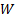, where the coefficients ofare the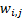referred to above. We can then write the formula deriving the output signals from the input signals as: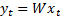We note that we have implicitly assumed a model of the world involving time homogeneity (or spatial homogeneity, if the signals involve a spatial dimension rather than a time dimension) i.e. that theand henceare constant through time.

If the mixing coefficients, i.e. the elements of, are known already then we can typically easily derive the input signals from the output matrix by inverting this matrix equation, i.e.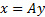where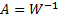.

However, we are more usually interested in the situation where the mixing coefficients are unknown (although as we have stated, we do implicitly assume with ICA that the mixture can be expressed in the above form, and that the corresponding coefficients are constant through time). We therefore seek an algorithm that estimates the unmixing coefficients, i.e. the coefficients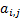of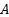, directly from the data, allowing us then to recover the signals themselves (and the original mixing coefficients).

We note that there is no way in such a framework of distinguishing between two input signals that are constant multiples of each other. Thus ICA and its variants will only generally identify signals up to a scalar multiplier (although we might in practice impose some standardised scaling criteria when presenting the answers or deciding which signals to retain and which to discard as ‘insignificant’ or unlikely to correspond to a true input signal).

If the number of input and output signals is the same then a matrix such ascan be viewed as corresponding to a vector transformation in an-dimensional vector space (being the number of input signals) spanned by vectors corresponding to the input signals. In this representation, each input signal would be characterised by a vector of unit length in the direction of a particular axis in this-dimensional space, with each different pair of input signals being orthogonal to each other (in geometric terms, ‘perpendicular’ to each other). Any possible (linear combination) mixture of these signals then corresponds to some vector in the same vector space, and a set ofof them corresponds to a set ofvectors in such a space. An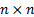matrix thus defines how simultaneously to map one set ofvectors to another in a way that respects underlying linear combinations. The types of transformations that are catered for by such matrices include rotation, shearing, and expansion away from or contraction towards the origin. Inverting such a matrix (if this is possible) corresponds to identifying the corresponding inverse transformation that returns a set oftransformed signal vectors to their original positions.

ICA uses this insight by identifying which (orthogonal) mixtures of the output series seem to exhibit the largest amount of ‘independence’, ‘non-normality’ or ‘lack of randomness’, since these mixtures can then be expected to correspond to the original input series (or scalar multiples of them). We note that not only will ICA not be able to differentiate between two signals that are scalar multiples of each other, but also that it won’t be able to differentiate between, say, two different pairs of signals in which the ordering of the signals is reversed. This is because it doesn’t directly include any prescription that ensures that any particular input signal will be mapped back to its own original axis. Instead, it is merely expected to arrange for each input signal to be mapped back to any one of the original (orthogonal) axes (but for no two different input signals to be mapped back onto the same axis). However, there may be a natural ordering that can be applied to the extracted signals, e.g. if input signals are expected to be strongly non-normal then we might order the extracted signals so that the first one is the most non-normal according to the relevant measure of non-normality that we have chosen, etc.